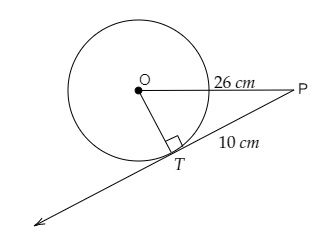# A point P is 26 cm away from the centre O of a circle and the length PT of the tangent drawn from P to the circle is 10 cm. Find the radius of the circle.

Given:

A point P is 26 cm away from the centre O of a circle and the length PT of the tangent drawn from P to the circle is 10 cm.

To do:

We have to find the radius of the circle.

Solution:$TP = 10\ cm$ and $OP = 26\ cm$

$PT$ is the tangent to the circle at $T$.

$OT\ perp\ PT$

In right angled triangle $OTP$,

By Pythagoras theorem,

$OP^2= OT^2+ TP^2$

$26^2 = OT^2 + 10^2$

$OT^2= 676 - 100$

$= 576$

$= 24^2$

Therefore,

$OT = 24\ cm$

The length of the radius of the circle is 24 cm.

Updated on: 10-Oct-2022

55 Views